• 参数方程 公式角度 x=r(θ)cosθx=r(\theta)cos\thetax=r(θ)cosθ y=r(θ)sinθy=r(\theta)sin\thetay=r(θ)sinθ 形象化 由于很值得注意的是： r(θ)→r(θ+dθ)​r(\theta) \rightarrow r(\theta+d\theta)​r(θ)...
参数方程
公式角度
$x=r(\theta)cos\theta$
$y=r(\theta)sin\theta$
$x^{\prime}=\dot{r}cos\theta-rsin\theta$
$y^{\prime}=\dot{r}sin\theta+rcos\theta$
$(x^{\prime})^2+(y^{\prime})^2=[r(\theta)]^2+[r^{\prime}(\theta)]^2$
形象化
由于很值得注意的是：
$r(\theta) \rightarrow r(\theta+d\theta)​$
$r(\theta+d\theta)-r(\theta)​$
$=\frac{r(\theta+d\theta)-r(\theta)}{d\theta}d\theta​$
$=\dot{r}d\theta​$
$\triangle r=\sqrt{{(\dot{r}d\theta)}^2+(rsind\theta)^2}$
我们注意$d\theta\approx0,sind\theta=d\theta$
$\triangle r=\sqrt{\dot{r}^2+r^2}d\theta$


展开全文• 参数方程中参数的意义： 参数方程中t的几何意义要看具体的曲线方程了，一般都是长度，角度等几何量，也有一些是不容易找到对应的几何量的。 参数方程定义： 一般的，在平面直角坐标系中，如果曲线上任意一点的...
目录

参数方程中参数的意义：

参数方程定义：

什么是参数方程：

参数方程与普通方程的公式：

举例：

参数方程：

参数方程中参数的意义：

参数方程中t的几何意义要看具体的曲线方程了，一般都是长度，角度等几何量，也有一些是不容易找到对应的几何量的。

参数方程定义：

一般的，在平面直角坐标系中，如果曲线上任意一点的坐标x，y都是某个变数t的函数{x=f(t)，y=g(t)并且对于t的每一个允许值，由上述方程组所确定的点M（x,y)都在这条曲线上，那么上述方程则为这条曲线的参数方程，联系x，y的变数t叫做变参数，简称参数，相对于参数方程而言，直接给出点的坐标间关系的方程叫做普通方程。

什么是参数方程：

其实就是 ：

y=f（t）；x=g（t）；其中t是参数，分别能表示出x，y；你看看下面参数方程与一般函数的转化你就明白了；

参数方程与普通方程的公式：

参数方程与普通方程的互化最基本的有以下四个公式：

1.cos²θ+sin²θ=1

2.ρ=x²+y²

3.ρcosθ=x

4.ρsinθ=y

举例：

参数方程：

一般的参数方程，主要使2式子进行乘除运算消掉  t。

遇到三角三角函数一般使用公式带入，消掉。

x=3-2t ①
y=-1－4t ②

解：
①×2-②得
x-2y=2(3-2t)-(-1-4t)
x-2y=7
∴2x-y = 7

将x， y的中参数转化为同一的，之后进行替换，得出一般函数方程。

例子：

x=cosθ （θ为参数） ①
y=cos2θ+1 ②
由②得
y=2cos²θ-1+1
y=2cos²θ
由①得
cosθ=x
∴y=2x² -1

例：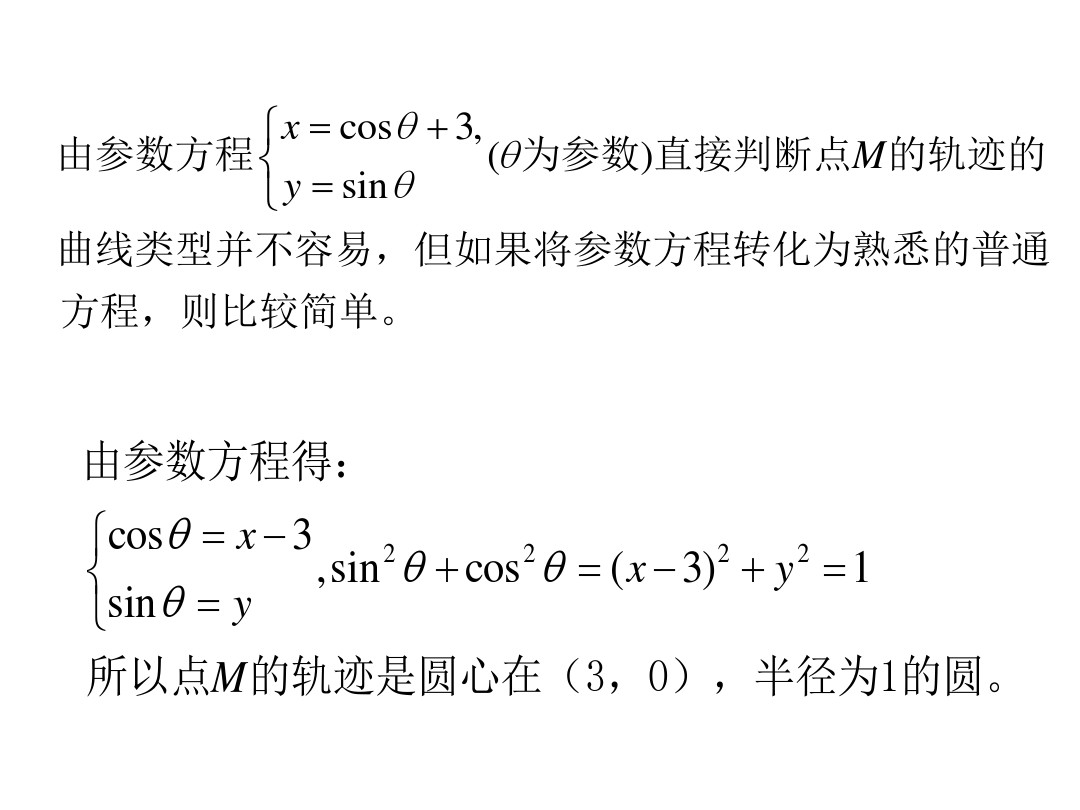又例圆，椭圆等：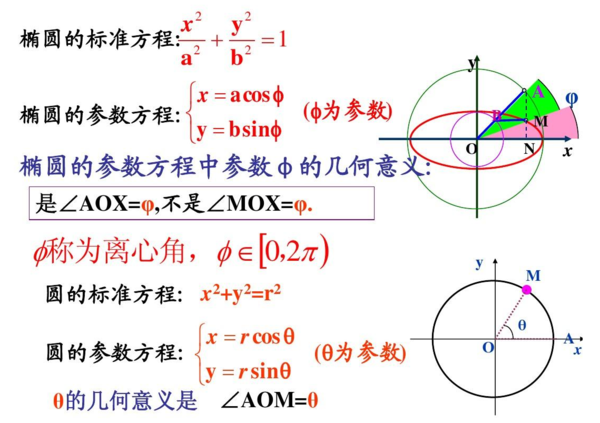展开全文• 这没有什么新东西，也没有进行计算。 我收集了各种参数方程并绘制了它们。 方程式显示在标题中。 按下一步转到下一个情节。 我已经包括了我知道的所有方程式，如果有任何有趣的事情请评论； 我将包含在文件中...matlab
• 椭圆的参数方程，以及相关例题解析。椭圆标准方程和参数方程的关系椭圆
• 2. 参数方程的概念 （一元函数y=f(x)，方程F(x,y)=0的图形通常为平面曲线） 3. 竖直判断法判断图形是否为函数图形 4. 曲线的参数方程 5. 直角坐标方程化为参数方程 6. 摆线 7. ...


1. 引例（如何描述动点的轨迹？）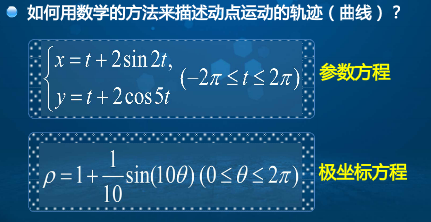2. 参数方程的概念 （一元函数y=f(x)，方程F(x,y)=0的图形通常为平面曲线）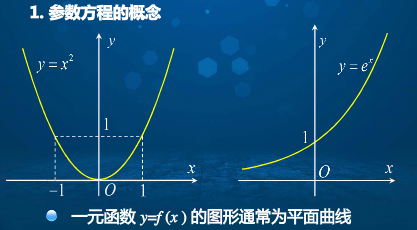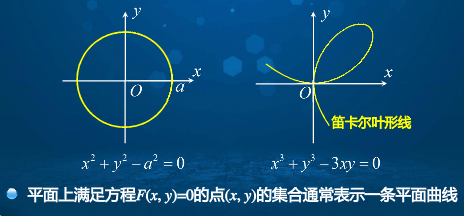3. 竖直判断法判断图形是否为函数图形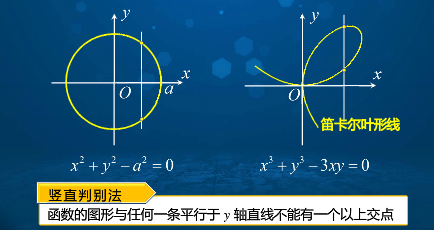4. 曲线的参数方程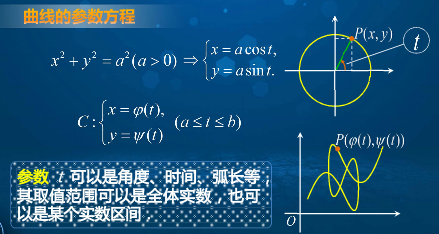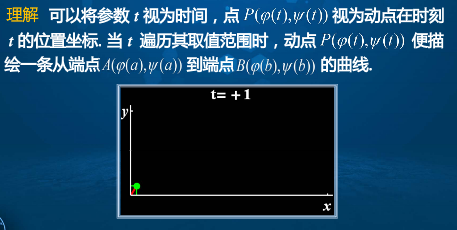5. 直角坐标方程化为参数方程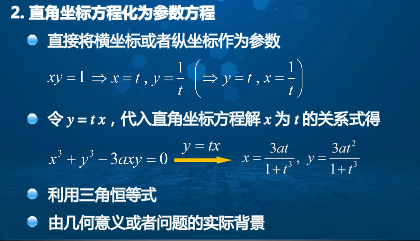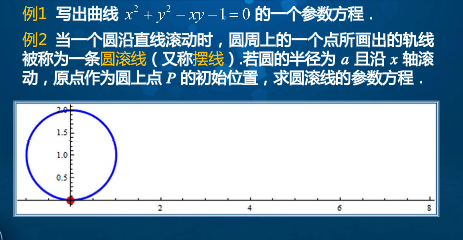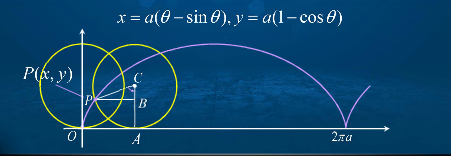6. 摆线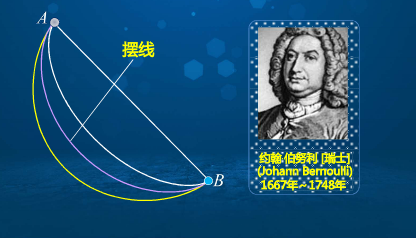7. 常见曲线的参数方程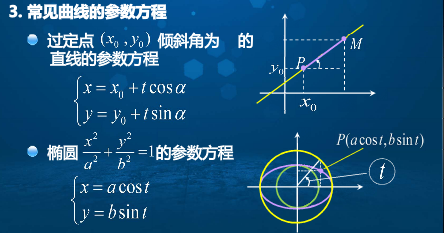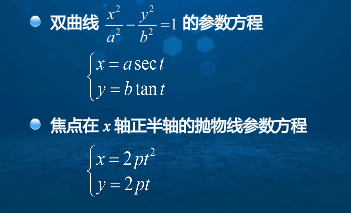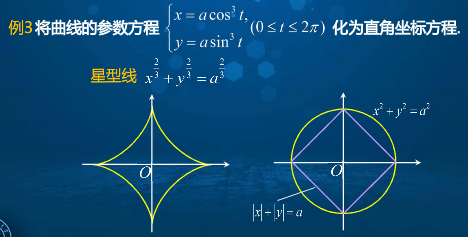展开全文• 对数直线参数方程的标准形式及一般形式中的参数进行了探讨,明确了各种情况下参数的几何意义,并例析参数几何意义的应用．
• 给出一种空间圆周的参数方程描述，便于计算机编程实现。
• 文章目录平面的参数方程平面的向量式方程平面的行列式方程平面的三点式方程平面的点法式方程平面的一般方程平面的一般方程讨论小结：平面方程的几种形式参考资料 平面是随处可见的空间形状 问题1：如何从几何上确定...
文章目录平面的参数方程平面的向量式方程平面的行列式方程平面的三点式方程平面的点法式方程平面的一般方程平面的一般方程讨论小结：平面方程的几种形式参考资料

06向量及其坐标表示、向量的方向角与方向余弦、向量组共线与共面的条件、向量的加法与数乘运算、向量组的线性组合、二维向量的基向量分解、三维向量的基向量分解、用坐标做向量的数乘
07向量的点积、数量积、两向量垂直的条件、投影与投影向量、向量的正交分解、几个不等式、用坐标计算数量积
08向量的叉积、向量积、用坐标行列式计算向量积、二重外积
09向量的混合积、向量之间的位置关系、用坐标行列式计算混合积、三向量共面的条件
10空间直线方程、参数方程、向量式方程、点向式方程、两点式方程、一般方程、空间直线的一般方程化为点向式方程
11空间平面方程、参数方程、向量式方程、行列式方程、三点式方程、点法式方程、一般方程

平面是随处可见的空间形状问题1：如何从几何上确定一个平面？不在一条直线上的三点确定一个平面 ．
过一定点且垂直于一定直线可以作一个平面．一条直线和直线外一点确定一个平面．
两相交直线确定一个平面．
两平行直线确定一个平面．

问题2：如何从代数上描述一个平面 ？
用代数方程式刻画平面上动点的轨迹，即建立平面的方程 .
在直角坐标系下，建立动点坐标满足的方程式 .
平面的参数方程
不在一条直线上的三点确定一个平面
要素：点 $M_{0}\left(x_{0}, y_{0}, z_{0}\right),$ 不共线的向量 $\boldsymbol{a}, \boldsymbol{b}$  （已知要素）
任务：求平面$\pi$  的方程即动点$𝑀(𝑥, 𝑦, 𝑧)$的轨迹方程．
依据： $\overrightarrow{M_{0} M}$与不共线的向量 𝒂 , 𝒃 共面 .关系式：
$\large{\color{red}{\overrightarrow{M_{0} M}=\lambda \boldsymbol{a}+\mu \boldsymbol{b}}}$
$\boldsymbol{a}=\left(a_{1}, a_{2}, a_{3}\right),\boldsymbol{b}=\left(b_{1}, b_{2}, b_{3}\right)$  得到：
$\boldsymbol{\pi}:\left\{\begin{array}{l}x=x_{0}+\lambda a_{1}+\mu b_{1} \\ y=y_{0}+\lambda a_{2}+\mu b_{2} \\ z=z_{0}+\lambda a_{3}+\mu b_{3}\end{array}\right.\tag1$
称为平面 $\pi$ 的参数方程 $.$其中 $\lambda, \mu$ 为参数。

平面上的点都满足方程 。
满足方程的点都在平面上， 不在平面上的点不满足方程 .

平面的向量式方程$\large{\color{red}{\overrightarrow{M_{0} M}=\lambda \boldsymbol{a}+\mu \boldsymbol{b}}}$  ，由
$r=\overrightarrow{O M}=(x,y,z)\\ r_0=\overrightarrow{O M_0}=(x_0,y_0,z_0)$
得到：$\quad \pi: r=r_{0}+\lambda a+\mu b$.
称为平面的向量式方程.
平面的行列式方程
要素：点 $M_{0}\left(x_{0}, y_{0}, z_{0}\right),$ 不共线的向量 $\boldsymbol{a}, \boldsymbol{b}$  （已知要素）
依据：三向量 $\overrightarrow{M_{0} M}, \boldsymbol{a}, \boldsymbol{b} \quad$ 共面,则它们的混合积为 0 .
平面 $\pi$ 的行列式方程为
$\left|\begin{array}{ccc} x-x_{0} & y-y_{0} & z-z_{0} \\ a_{1} & a_{2} & a_{3} \\ b_{1} & b_{2} & b_{3} \end{array}\right|=0$
平面的三点式方程
要素： 不在一条直线上的三点
$M_{1}\left(x_{1}, y_{1}, z_{1}\right), M_{2}\left(x_{2}, y_{2}, z_{2}\right), M_{3}\left(x_{3}, y_{3}, z_{3}\right)$
$\text { 依据：三向量 } \overrightarrow{M_{1} M}, \overrightarrow{M_{1} M_{2}}, \overrightarrow{M_{1} M_{3}} \text { 共面 } .$$\text { 平面 } \pi \text { 的方程为 }$
$\begin{array}{l} \left|\begin{array}{ccc} x-x_{1} & y-y_{1} & z-z_{1} \\ x_{2}-x_{1} & y_{2}-y_{1} & z_{2}-z_{1} \\ x_{3}-x_{1} & y_{3}-y_{1} & z_{3}-z_{1} \end{array}\right|=0 \end{array}$
一称为平面 $\pi$ 的三点式方程
平面的点法式方程
过一定点且垂直于一定直线可以作一个平面．
平行于定直线的非零向量是垂直于平面的，称为平面的法向量.
要素：点 $M_{0}\left(x_{0}, y_{0}, z_{0}\right),$ 法向量 $n$ 。

任何垂直于平面的非零向量  $n$ 都是平面的法向量．
与 $n$平行的所有非零向量均可作为此平面的法向量．
平面上的所有向量都与该平面的法向量垂直．要素：点 $M_{0}\left(x_{0}, y_{0}, z_{0}\right),$ 法向量 $\boldsymbol{n}=(A, B, C)$.
设 $M(x, y, z)$ 为平面上的动点, 则
$\boldsymbol{n} \perp \overrightarrow{M_{0} {M}} ,~~~~~~ 向量关系$
$\begin{array}{lll}\text { 有 } & \boldsymbol{n} \cdot \overrightarrow{\boldsymbol{M}_{0} \boldsymbol{M}}=\mathbf{0} . & \text { 向量代数关系 }\end{array}$
平面 $\pi$ 的点法式方程 $.$ :
$\pi: A\left(x-x_{0}\right)+B\left(y-y_{0}\right)+C\left(z-z_{0}\right)=0$
平面的一般方程
点法式  ： $\pi: A\left(x-x_{0}\right)+B\left(y-y_{0}\right)+C\left(z-z_{0}\right)=0$  ,转化得到
$\pi: A x+B y+C z+D=0$
称为平面 $\pi$ 的一般方程,其中 $n=(A, B, C)$ 为平面的法向量
【注】 平面方程是一个三元一次方程 .反之，一个三元一次方程在几何上表示一个平面 .
平面的一般方程讨论
$\pi: A x+B y+C z+D=0 \quad(A, B, C$ 不全为0 $)$
(1) 当 $D=0$ 时, 平面 $\pi: A x+B y+C z=0$ 过原点;
(2) 当 $A=0$ 时, 平面 $\pi: B y+C z+D=0$ 平行于 $x$ 轴;
当 $B=0$ 时, 平面 $\pi: A x+C z+D=0$ 平行于 $y$ 轴
当 $C=0$ 时, 平面 $\pi: A x+B y+D=0$ 平行于 $z$ 轴
(3) 当 $A=0, D=0$ 时, 平面 $\pi: B y+C z=0$ 过 $x$ 轴;
当 $B=0, D=0$ 时, 平面 $\pi: A x+C z=0$ 过 $y$ 轴;
当 $C=0, D=0$ 时, 平面 $\pi: A x+B y=0$ 过 $z$ 轴
(4) 当 $A=0, B=0$ 时, 平面 $\pi: C z+D=0$ 平行于 $x O y$ 面
当 $B=0, C=0$ 时, 平面 $\pi: A x+D=0$ 平行于 $y O z$ 面
当 $A=0, C=0$ 时，平面 $\pi: B y+D=0$ 平行于 $z O x$ 面.
$\Large\color{violet}{例1}$   求过 $M_{0}(2,1,1)$ 且平行于 $\pi_{1}: x+2 y-3 z+7=0$ 的平面方程
【解法一】 因所求平面 $\pi$ 与已知平面 $\pi_{1}$ 平行, 则 $\pi_{1}$ 的法向量
$n=(1,2,-3)$
也是 $\pi$ 的法向量，则平面 $\pi$ 的点法式方程为
$1 \cdot(x-2)+2 \cdot(y-1)+(-3) \cdot(z-1)=0$
整理得平面 $\pi$ 的一般方程为
$x+2 y-3 z-1=0$
【解法二 】因所求平面 $\pi$ 与已知平面 $\pi_{1}$ 平行, 设平面 $\pi$ 的一般方程为
$x+2 y-3 z+D=0$
代入点 $M_{0}(2,1,1)$ 的坐标, 得 $2+2-3+D=0, \quad$ 故 $D=-1 .$
于是平面 $\pi$ 的方程为
$x+2 y-3 z-1=0$
$\Large\color{violet}{例2}$  已知一平面 $\pi$ 与三个坐标轴的交点分别为
$P(a, 0,0), \quad Q(0, b, 0), \quad R(0,0, c) \quad(a, b, c \neq 0)$
求平面 $\pi$ 的方程 .
【解法一】由平面的三点式方程知
$\left|\begin{array}{ccc} x-a & y-0 & z-0 \\ 0-a & b-0 & 0-0 \\ 0-a & 0-0 & c-0 \end{array}\right|=0$
整理得平面 $\pi$ 的一般方程为 $\quad b c x+a c y+a b z-a b c=0 .$
进一步整理得 $\quad \pi: \frac{x}{a}+\frac{y}{b}+\frac{z}{c}=1 . \quad \longrightarrow$ 称为平面 $\pi$ 的$\large\color{#70f3ff}{\boxed{\color{green}{截距式方程}}}$
【解法二 】 设平面 $\pi$ 的一般方程为 $\pi: A x+B y+C z+D=0,$ 则
$\left\{\begin{array}{l} A a+D=0 \\ B b+D=0 \\ C c+D=0 \end{array}\right.$
解得 $A=-\frac{D}{a}, B=-\frac{D}{b}, C=-\frac{D}{c} .$ 因 $A, B, C$ 不全为 $0,$ 则 $D \neq 0$. 于是得
$\quad \pi: \frac{x}{a}+\frac{y}{b}+\frac{z}{c}=1$
$\Large\color{violet}{例3}$   已知空间四点 $A(1,0,0), B(2,1,3), C(1,2,5), P(2,3, t)$
问：当 $t$ 为何值时, 这四点在一个平面上? 并求出该平面方程.
【解 】$A(1,0,0), B(2,1,3), C(1,2,5), P(2,3, t)$ 四点共面的充要条件是三向量 $\overrightarrow{A P}=(1,3, t), \overrightarrow{A B}=(1,1,3), \overrightarrow{A C}=(0,2,5)$ 共面, $\quad$ 故
$\left|\begin{array}{lll} 1 & 3 & t \\ 1 & 1 & 3 \\ 0 & 2 & 5 \end{array}\right|=2 t-16=0, \text { 即 } t=8$
所以, 当 $t=8$ 时, $A 、 B 、 C 、 P$ 四点共面.
该平面的三点式方程为
$\left|\begin{array}{ccc} x-1 & y-0 & z-0 \\ 2-1 & 1-0 & 3-0 \\ 1-1 & 2-0 & 5-0 \end{array}\right|=\left|\begin{array}{ccc} x-1 & y & z \\ 1 & 1 & 3 \\ 0 & 2 & 5 \end{array}\right|=0$
整理得平面的一般方程为 $x+5 y-2 z-1=0$.
$\Large\color{violet}{注：}$也可以先求出由 $A 、 B 、 C$ 三点所确定的平面的方程
$x+5 y-2 z-1=0$
再代入点P的坐标，得 $t=8$.
小结：平面方程的几种形式
设点 $M_{0}\left(x_{0}, y_{0}, z_{0}\right)$ 在平面上, $\quad \boldsymbol{n}=(A, B, C)$ 是平面的法向量
1、平面的点法式方程
$A\left(x-x_{0}\right)+B\left(y-y_{0}\right)+C\left(z-z_{0}\right)=0$
2、平面的一般式方程
$A x+B y+C z+D=0$
3 、平面的截距式方程
$\frac{x}{a}+\frac{y}{b}+\frac{z}{c}=1 . \quad$ 其中 $a, b, c$ 为平面在三个坐标轴上的截距.
4 、平面的三点式方程
设 $M_{1}\left(x_{1}, y_{1}, z_{1}\right), M_{2}\left(x_{2}, y_{2}, z_{2}\right), M_{3}\left(x_{3}, y_{3}, z_{3}\right)$ 是平面上不共线的三点, 则
$\left|\begin{array}{ccc} x-x_{1} & y-y_{1} & z-z_{1} \\ x_{2}-x_{1} & y_{2}-y_{1} & z_{2}-z_{1} \\ x_{3}-x_{1} & y_{3}-y_{1} & z_{3}-z_{1} \end{array}\right|=0$
5 、平面的参数式方程 $\quad \pi:\left\{\begin{array}{l}x=x_{0}+\lambda a_{1}+\mu b_{1} \\ y=y_{0}+\lambda a_{2}+\mu b_{2}, \\ z=z_{0}+\lambda a_{3}+\mu b_{3}\end{array}\right.$
6 、平面的行列式方程 $\quad\left|\begin{array}{ccc}x-x_{0} & y-y_{0} & z-z_{0} \\ a_{1} & a_{2} & a_{3} \\ b_{1} & b_{2} & b_{3}\end{array}\right|=0$.
7 、平面的向量式方程 $\quad \pi: r=r_{0}+\lambda a+\mu b$.
参考资料
空间解析几何_国防科技大学
北京大学数学系几何与代数教研室代数小组，《高等代数》（第四版）
高等代数，林亚南，高等教育出版社
高等代数学习辅导，林亚南，林鹭，杜妮，陈清华，高等教育出版社
高等代数    电子科技大学
高等代数_安阳师范学院
《高等代数》（第五版）


展开全文• ## matlab参数方程拟合

千次阅读 2019-08-30 22:12:13
Matlab参数方程拟合拟解决的问题：问题描述数据程序结论 拟解决的问题： 假设已经得到了一个描述特定数据规律的方程，但是不知道方程参数的具体值，通过与已有数据的拟合求解方程参数。 以种群增长模型为例—— 问题...matlab 数学建模
• ## 图解圆的参数方程

万次阅读 2018-06-15 17:11:02
• 以入射光束"孔径高度"为参数,根据等光程原理,通过光线追迹法建立了参数和坐标点(两个分量)一一对应的关系式,称为等光程参数方程。在该方程中,参数是连续的实变量,参数方程给出的曲线是连续的实线,即非球面子午截线,...
• ## 直线参数方程推导

万次阅读 2018-11-07 23:39:28
今天复习一个知识点的时候涉及到了参数方程这个概念，然后发现自己基本完全忘光了，因此特此记录一下。 我们先来回忆一下直线的普通方程有哪些： 点斜式 已知直线 l 的斜率是 k，并且经过点 P(x0,y0)P(x_0, y_0)P...
• 参数方程与极坐标问题引入曲线的参数方程曲线参数方程直角坐标方程化为参数方程例1 方法三，利用三角恒等式例2，方法四，几何背景常见的参数方程如何把直角坐标方程转化为参数方程极坐标与极坐标方程直角坐标系与极...算法
• 给出了椭圆隐式方程和参数方程的互相转化，并通过MATLAB程序进行验证，实验证明了转换关系的正确性。线性代数 矩阵
• 高二数学选修4-4曲线的参数方程，高二数学选修4-4曲线的参数方程课件，高二数学选修4-4曲线的参数方程PPT
• 一、曲线的参数方程 1.1 参数方程的概念 一般地，在平面直角坐标系中，如果曲线上任意一点的坐标x,yx,yx,y都是某个变数ttt的函数 {x=f(t)y=g(t)(1) \left\{ \begin{aligned} &x=f(t)\\ &y=g(t) \end{aligned...
• 文章目录空间直线的参数方程空间直线的向量式方程直线的点向式方程空间直线的两点式方程空间直线的一般方程空间直线的一般方程化为点向式方程小结：直线方程的几种形式参考资料 直线是随处可见的空间形状 问题:如何...
• 2022届一轮复习北师大版 极坐标方程与参数方程 课件（39张）.pptx
• 首先推导和证明了空间任意平面上圆的参数方程，在此基础上给出了沿空间任意方向圆柱面的参数方程及其在计算机绘制的算法，还进一步将结果推广到空间任意方向圆锥面、椭圆柱面参数方程的建立并给出了相应的结果。...
• 积分域边界曲线为参数方程的二重积分的计算 1，此题来源于李永乐复习全书(数学一,2018版本)二重积分章节的某一例题，提出了在计算二重积分的过程中，当积分域的边界曲线为参数方程时的二重积分的一种常用解法思想...高等数学 二重积分
• 2022届一轮复习北师大版 极坐标方程与参数方程的应用 课件（24张）.pptx
• Matlab使用杂谈2-参数方程转换参数方程转换solve函数使用参数方程转换使用实例 参数方程转换 在进行数学建模时，有时候我们会得到两个具有相同变量的方程，但是如何求除这两个方程的因变量之间的关系，此时就可以...matlab 算法 函数式编程
• 1.圆的参数方程表示形式 Python代码： import numpy as np import matplotlib.pyplot as plt # 1.圆半径 r = 2.0 # 2.圆心坐标 a, b = (0., 0.) # 参数方程 theta = np.arange(0, 2*np.pi, 0.01) x = a + ...
• 2022届一轮复习北师大版 参数方程 学案.doc...